Courses

# Test: Complex Number (Competition Level) - 1

## 30 Questions MCQ Test Mathematics (Maths) Class 11 | Test: Complex Number (Competition Level) - 1

Description
This mock test of Test: Complex Number (Competition Level) - 1 for JEE helps you for every JEE entrance exam. This contains 30 Multiple Choice Questions for JEE Test: Complex Number (Competition Level) - 1 (mcq) to study with solutions a complete question bank. The solved questions answers in this Test: Complex Number (Competition Level) - 1 quiz give you a good mix of easy questions and tough questions. JEE students definitely take this Test: Complex Number (Competition Level) - 1 exercise for a better result in the exam. You can find other Test: Complex Number (Competition Level) - 1 extra questions, long questions & short questions for JEE on EduRev as well by searching above.
QUESTION: 1

### The locus of the point z = x + iy satisfying the equation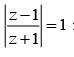is given by

Solution: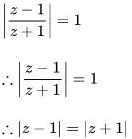i.e. lie on the ⊥ bisector the line joining (1,0) & (-1,0)
i.e. the y axis
∴ 2 lies on x = 0

QUESTION: 2

### If  Arg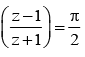then the locus of z is

Solution:

s of z is a circle having (–1, 0) and (1, 0) as its ends of diameter.

QUESTION: 3

### In Argand diagram all the complex numbers z satisfying |z - 4i + z + 4i = 10| lie on a

Solution:

|z1 - z2| = |4i + 4i| = 8 < 10
∴Then locus of z is ellipse.

QUESTION: 4

If z is a complex number, then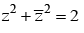represents

Solution:

Let z = x + iy, then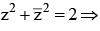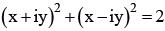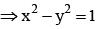QUESTION: 5

If xn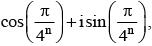then x1, x2, x3, .....∞

Solution: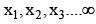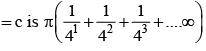QUESTION: 6

If w is a complex cube root of unity then the value of (1 + ω) (1 + ω​)2 (1 + ω4​) (1 + ω8​)...2n terms =

Solution:

Put n = 1 and verify

QUESTION: 7

If x2 + x + 1 = 0, then the value of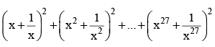is

Solution:

x = ω or ω2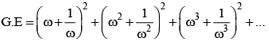= (1+1+4)9 = 54

QUESTION: 8

f |z - 2 + 2i| = 1, then the least value of |z| is

Solution: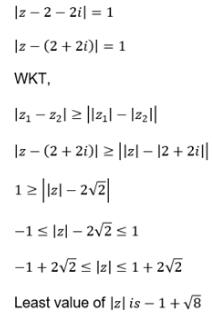QUESTION: 9

The maximum value of 3z + 9- 7| if |z + 2 - i| = 5 is

Solution:

|3z + 6 - 3i + 3 - 4i| |≤| 3z + 6 - 3i| + |3 - 4i| ≤ 3 |z + 2-i| + |3- 4i|
=15+5 = 20

QUESTION: 10

The value of  log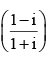is

Solution:
QUESTION: 11

If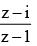is purely imaginary then the locus of z is

Solution:

Let z = x + iy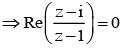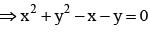QUESTION: 12

If zr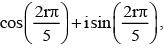where r = 1,2,3,4,5 then z1z2z3z4z5 =

Solution:
QUESTION: 13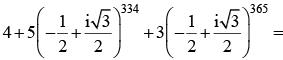Solution:

4 + 5ω334 + 3ω365 = 4 + 5ω + 3ω2
=1+ 2ω+3 (1+ω+ω2) =1+ 2ω = i √3

QUESTION: 14

For all complex numbers z1z2 such that |z1| = 12 and |z2 - 3 - 4i| = 5 the  minimum value of |z1 -z2| is

Solution: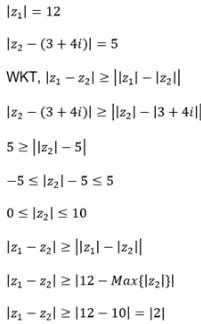QUESTION: 15

If a and b are real numbers between 0 and 1 such that the points z1 = a + i, z2 = 1 + bi and z3 =0 form an equilateral triangle then a, b are

Solution: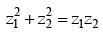and simplify

QUESTION: 16

If α,β are the roots of x2 - 2x + 4 = 0 then αn + βn =

Solution: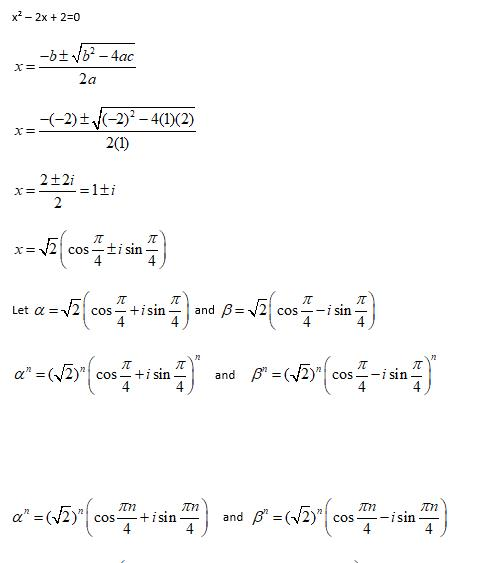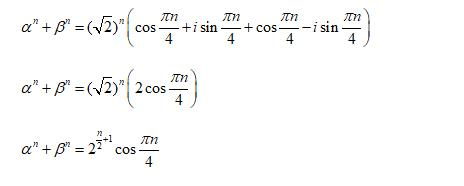QUESTION: 17

The value of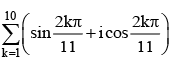is

Solution: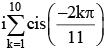= i[sum of roots -1] = i (0 - 1) = -i

QUESTION: 18

If the cube roots of unity are 1, ω,ω2 , then the roots of the equation ( x - 1)3 + 8 = 0 are

Solution: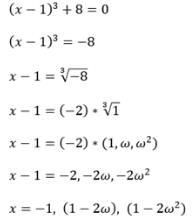QUESTION: 19

If |z1| = 2, |z2| = 3, |z3| = 4 and |z1 + z2 + z3| = 5 then |4z2z3 + 9z3z1 + 16z1z2| =

Solution:
QUESTION: 20

Let z = x + iy be a complex number where x and y are integers then the area of the rectangle whose vertices are the roots of the equation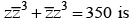Solution: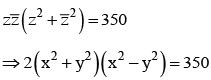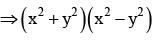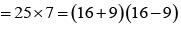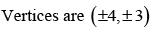QUESTION: 21

If a + ib =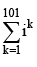, then (a, b) equals

Solution:

a + ib = i + (i2 + i3 + i4 + i5) + (i6 + i7 + i8 + i9) +....+ (i98 + i99 + i100 + i101)
a + ib = 0 + i  ⇒ a = 0, b = 1

QUESTION: 22

If z ∈ C and 2z =| z |+i, then z equals

Solution: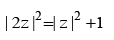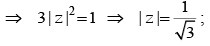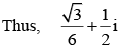QUESTION: 23

Suppose,  a, b, c ∈ C , and | a |=| b |=| c |= 1 and abc  = a +b + c, then bc +ca + ab is equal to

Solution: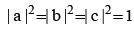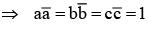Now, abc = a + b + c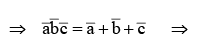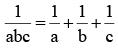⇒ bc + ca + ab = 1

QUESTION: 24

The number of complex numbers z which satisfy z2 + 2 | z |2 = 2 is

Solution:

As z2 = 2(1 -|z|2) is real, z is either purely and or purely imaginary.

If z is purely real, then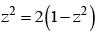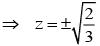In this case,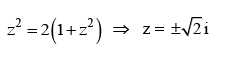QUESTION: 25

The complex numbers sin x + i cos 2x and cos x - i sin 2x are conjugate to each other for

Solution: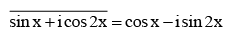⇒ sin x - i cos 2x = cos x - i sin 2x
⇒ sin x = cos x and    cos 2x = sin 2x
⇒ tan x = 1 and tan 2x = 1
These two equations cannot hold simultaneously.

QUESTION: 26

If z1 and z2 are two complex numbers are a, b are two real numbers,then |az1 - bz2|2 + |bz1 + az2|2 equals

Solution:

We have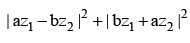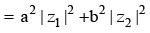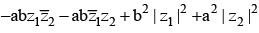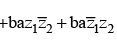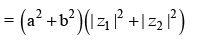QUESTION: 27

If z ≠ 0 is a complex number such that arg(z) = π/4,then

Solution:

As arg(z) =π/4, we can write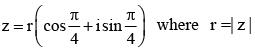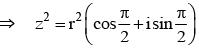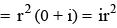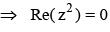QUESTION: 28

Let z and w be two non-zero complex numbers such that |z| = |w| and arg (z) + arg (w) = π. Then z is equal to

Solution:

Let |z| = |w| = r and arg(w) = θ, so that arg (z) = π - θ

We have,  z = r  [cos(π - θ)]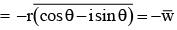QUESTION: 29

If z1, z2, z3 are complex numbers such that

|z1| = |z2| = |z3| =|1/z1 +1/z2 + 1/z3| = 1, then find the value of  z1+ z2 + z3 .

Solution: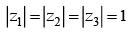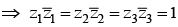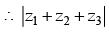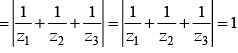QUESTION: 30

If (x + iy)1/3 = a + ib, then  x/a + y/b  equals

Solution:

(x + iy)1/3 = a + ib
⇒ x + iy = (a3 – 3ab2) + (3a2b – b3)I
⇒ x/a = a2  - b2
Thus x/a + y/b = 4a2 - 4b2